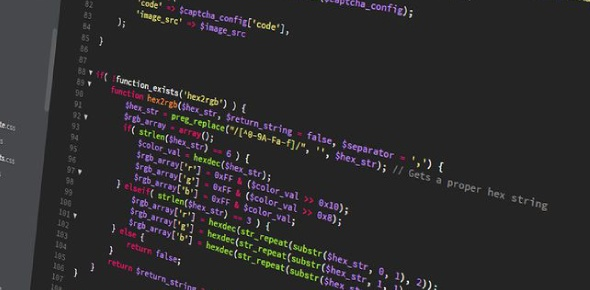# Test Your Python Skills! Hardest Trivia Questions Quiz

35 Questions | Attempts: 310
ShareSettings• 1.
Please select the logo of Python in the given image.
• 2.
Which is the correct operator for power(x^y)?
• A.

X^y

• B.

X**y

• C.

X^^y

• D.

None of the mentioned

• 3.
Which one of these is floor division?
• A.

/

• B.

//

• C.

%

• D.

None of the mentioned

• 4.
What is answer of this expression, 22 % 3 is?
• A.

7

• B.

1

• C.

0

• D.

5

• 5.
You can perform mathematical operation on String?
• A.

True

• B.

False

• 6.
Operators with the same precedence are evaluated in which manner?
• A.

Left to Right

• B.

Right to Left

• 7.
What is the output of this expression, 3*1**3?
• A.

27

• B.

9

• C.

3

• D.

1

• 8.
Which one of the following have the same precedence?
• A.

• B.

Multiplication and Division

• C.

Both the options

• D.

None of the options

• 9.
Int(x) means variable x is converted to integer.
• A.

True

• B.

False

• 10.
Which one of the following have the highest precedence in the expression?
• A.

Exponential

• B.

• C.

Multiplication

• D.

Parentheses

• 11.
What is returned by math.ceil(3.4)?
• A.

3

• B.

4

• 12.
What is the value returned by math.floor(3.4)?
• A.

3

• B.

4

• 13.
What is the value returned by math.fact(6)?
• A.

720

• B.

6

• C.

[6,5,4,3,2,1]

• D.

Error

• 14.
Which of the following statement prints hello\example\test.txt ?
• A.

Print(“hello\example\test.txt”)

• B.

Print(“hello\\example\\test.txt”)

• C.

Print(“hello\”example\”test.txt”)

• D.

Print(“hello”\example”\test.txt”)

• 15.
Suppose s is “\t\tWorld\n”, what is s.strip() ?
• A.

\t\tWorld\n

• B.

\tWorld\n

• C.

\t\tWORLD\n

• D.

World

• 16.
What is the output of “hello”+1+2+3 ?
• A.

Hello123

• B.

Hello

• C.

Error

• D.

Hello6

• 17.
Say s=”hello” what will be the return value of type(s) ?
• A.

Int

• B.

Bool

• C.

Str

• D.

String

• 18.
Python is case sensitive when dealing with identifiers?
• A.

True

• B.

False

• 19.
What is the maximum possible length of an identifier?
• A.

31 characters

• B.

310 characters

• C.

255 characters

• D.

None of the mentioned

• 20.
Which of the following is invalid?
• A.

_a=1

• B.

__a = 1

• C.

A_a = 1

• D.

1 = _a

• 21.
Which of the following is an invalid variable?
• A.

My_string_1

• B.

1st_string

• C.

Foo

• D.

_a

• 22.
Which of the following cannot be a variable?
• A.

__init__

• B.

In

• C.

It

• D.

On

• 23.
Which of the following is the use of function in python?
• A.

Functions are reusable pieces of programs

• B.

Functions don’t provide better modularity for your application

• C.

You can’t also create your own functions

• D.

All of the mentioned

• 24.
Which keyword is use for function?
• A.

Fun

• B.

Def

• C.

Function

• D.

Define

• 25.
Which of these in not a core datatype?
• A.

Lists

• B.

Dictionary

• C.

Tuples

• D.

Class

## Related TopicsBack to top
×

Wait!
Here's an interesting quiz for you.